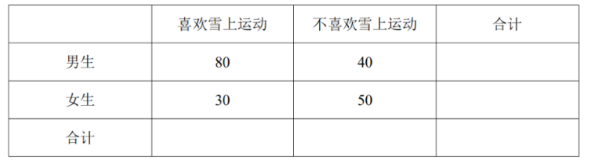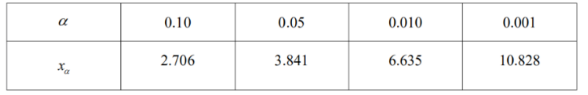2022 年北京冬奥会圆满落幕, 随后多所学校掀起了 “雪上运动” 的热潮. 为了 解学生对 “雪上运动” 的喜爱程度, 某学校从全校学生中随机抽取 200 名学生进行问卷调查, 得到以下数据:(1) 完成 $2 \times 2$ 列联表, 依据小概率值 $\alpha=0.01$ 的 $\chi^2$ 独立性检验, 能否认为是否喜欢雪上运 动与性别有关联?
(2) (i) 从随机抽取的这 200 名学生中采用分层抽样的方法抽取 20 人, 再从这 20 人中随机抽取 3 人. 记事件 $A=$ “至少有 2 名是男生”, 事件 $B=$ “至少有 2 名喜欢雪上运动的 男生”, 事件 $C=$ “至多有 1 名喜欢雪上运动的女生”. 试计算 $P(\mathrm{~A}) \cdot P(B \mid A) \cdot P(C \mid A B)$ 和 $P(A B C)$ 的值, 并比较它们的大小.
(ii) ( i ) 中 $P(A B C)$ 与 $P(\mathrm{~A}) \cdot P(B \mid A) \cdot P(C \mid A B)$ 的大小关系能否推广到更一般的情形? 请写出结论, 并说明理由.